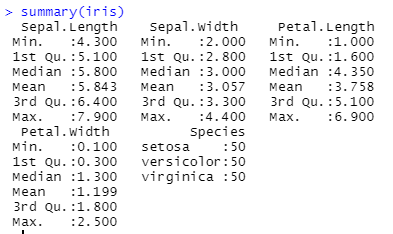top of page
Search
• Ekta Aggarwal

# Summary function in R

In this tutorial we will learn about how to get the 'summary' i.e., the descriptive statistics of various columns in a dataframe in R.

For this, we will make use of iris dataset, which is inbuilt in R. Let us firstly view the iris dataset using View command.

`View(iris)`iris has got information about 5 different attributes for 150 flowers. Thus, the shape of the dataset is 150 rows and 5 columns.

Let us use the summary function on our iris dataset. For this we write our object name inside the parenthesis ( ) of summary function.

`summary(iris)`

In the output below we can see that for numeric columns, summary functions returns the minimum, 1st quartile (i.e., 25th percentile), median (i.e., 50th percentile), mean, 3rd quartile (i.e., 75th percentile) and maximum. While for 'factor' columns(eg. Species) it returns the frequency distribution for each category.

Output:Now let us use the summary function on 'Species' column of iris dataset

`summary(iris\$Species)`

Please note that Species column has got character / factor values. Thus, in the output below we can see that it returns the frequency distribution for each flower Specie. i.e., Setosa, Versicolor and Virginica all 3 are appearing 150 times.

Output:Similarly, now let us use the summary function on 'Petal.Length' column of iris dataset

`summary(iris\$Petal.Length)`

Please note that 'Petal.Length' column has got numeric values.

In the output below we can see that summary functions is returning the minimum, 1st quartile (i.e., 25th percentile), median (i.e., 50th percentile), mean, 3rd quartile (i.e., 75th percentile) and maximum.

Output:See All
bottom of page